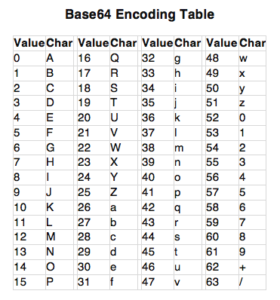# Encoding and Decoding Base64 Strings in Python

### Introduction

Have you ever received a PDF or an image file from someone via email, only to see strange characters when you open it? This can happen if your email server was only designed to handle text data. Files with binary data, bytes that represent non-text information like images, can be easily corrupted when being transferred and processed to text-only systems.

Base64 encoding allows us to convert bytes containing binary or text data to ASCII characters. By encoding our data, we improve the chances of it being processed correctly by various systems.

In this tutorial, we would learn how Base64 encoding and decoding works, and how it can be used. We will then use Python to Base64 encode and decode both text and binary data.

### What is Base64 Encoding?

Base64 encoding is a type of conversion of bytes into ASCII characters. In mathematics, the base of a number system refers to how many different characters represent numbers. The name of this encoding comes directly from the mathematical definition of bases – we have 64 characters that represent numbers.

The Base64 character set contains:

• 26 uppercase letters
• 26 lowercase letters
• 10 numbers
• `+` and `/` for new lines (some implementations may use different characters)

When the computer converts Base64 characters to binary, each Base64 character represents 6 bits of information.

Note: This is not an encryption algorithm, and should not be used for security purposes.

Now that we know what Base64 encoding and how it is represented on a computer, let’s look deeper into how it works.

### How Does Base64 Encoding Work?

We will illustrate how Base64 encoding works by converting text data, as it’s more standard than the various binary formats to choose from. If we were to Base64 encode a string we would follow these steps:

1. Take the ASCII value of each character in the string
2. Calculate the 8-bit binary equivalent of the ASCII values
3. Convert the 8-bit chunks into chunks of 6 bits by simply re-grouping the digits
4. Convert the 6-bit binary groups to their respective decimal values.
5. Using a base64 encoding table, assign the respective base64 character for each decimal value.

Let’s see how it works by converting the string “Python” to a Base64 string.

The ASCII values of the characters `P, y, t, h, o, n` are `15, 50, 45, 33, 40, 39` respectively. We can represent these ASCII values in 8-bit binary as follows:

`01010000 01111001 01110100 01101000 01101111 01101110`

Recall that Base64 characters only represent 6 bits of data. We now re-group the 8-bit binary sequences into chunks of 6 bits. The resultant binary will look like this:

`010100 000111 100101 110100 011010 000110 111101 101110`

Note: Sometimes we are not able to group the data into sequences of 6 bits. If that occurs, we have to pad the sequence.

With our data in groups of 6 bits, we can obtain the decimal value for each group. Using our last result, we get the following decimal values:

`20 7 37 52 26 6 61 46`

Finally, we will convert these decimals into the appropriate Base64 character using the Base64 conversion table:As you can see, the value `20` corresponds to the letter `U`. Then we look at `7` and observe it’s mapped to `H`. Continuing this lookup for all decimal values, we can determine that “Python” is represented as `UHl0aG9u` when Base64 encoded. You can verify this result with an online converter.

To Base64 encode a string, we convert it to binary sequences, then to decimal sequences, and finally, use a lookup table to get a string of ASCII characters. With that deeper understanding of how it works, let’s look at why would we Base64 encode our data.

### Why use Base64 Encoding?

In computers, all data of different types are transmitted as 1s and 0s. However, some communication channels and applications are not able to understand all the bits it receives. This is because the meaning of a sequence of 1s and 0s is dependent on the type of data it represents. For example, `10110001` must be processed differently if it represents a letter or an image.

To work around this limitation, you can encode your data to text, improving the chances of it being transmitted and processed correctly. Base64 is a popular method to get binary data into ASCII characters, which is widely understood by the majority of networks and applications.

A common real-world scenario where Base64 encoding is heavily used are in mail servers. They were originally built to handle text data, but we also expect them to send images and other media with a message. In those cases, your media data would be Base64 encoded when it is being sent. It will then be Base64 decoded when it is received so an application can use it. So, for example, the image in the HTML might look like this:

``````<img src="data:image/png;base64,aVRBOw0AKg1mL9...">
``````

Understanding that data sometimes need to be sent as text so it won’t be corrupted, let’s look at how we can use Python to Base64 encoded and decode data.